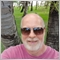# Why is Oncalculate() called twice with prev_calculated = 0 when client terminal starts up55

If you close the client terminal with a chart and indicator open and then re-open the client terminal, the chart with indicator will appear as expected. The indicator will receive its first call to OnCalculate with prev_calculated  = 0 as expected. However, on the next call OnCalculate will pass 0 a second time as the value of prev_calculated. What is the reason for this? This behaviour is not seen when an indicator is added to an already opened chart or when a template is added to a chart.Moderator
164455

robnorthen :

It has always been that way. When the terminal starts, the indicator can return prev_calculated = 0 several times.20105

1. Terminal starts.
2. Indicators/EAs are loaded. Static and globally declared variables are initialized. (Do not depend on a specific order.)
3. OnInit is called.
4. For indicators OnCalculate is called with any existing history.
5. Human may have to enter password, connection to server begins.
6. New history is received, OnCalculate called again.
7. New tick is received, OnCalculate/OnTick is called. Now TickValue, TimeCurrent, account information and prices are valid.Moderator
19401

robnorthen:
If you close the client terminal with a chart and indicator open and then re-open the client terminal, the chart with indicator will appear as expected. The indicator will receive its first call to OnCalculate with prev_calculated  = 0 as expected. However, on the next call OnCalculate will pass 0 a second time as the value of prev_calculated. What is the reason for this? This behaviour is not seen when an indicator is added to an already opened chart or when a template is added to a chart.

It is, in fact, very useful.

When prev_calculated ==0 you know that missing bars may have been updated and so the indicator will calculate all bars afresh (if it is coded to do so).38391

Keith Watford: